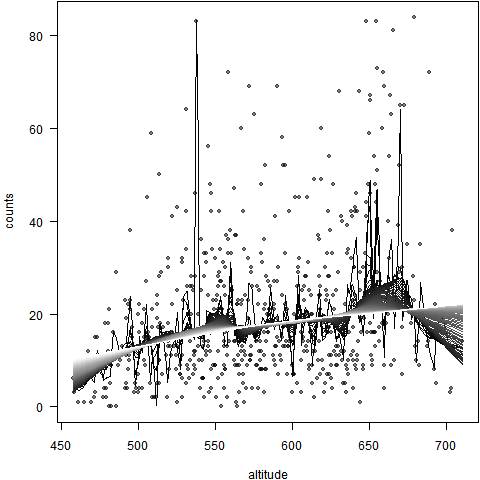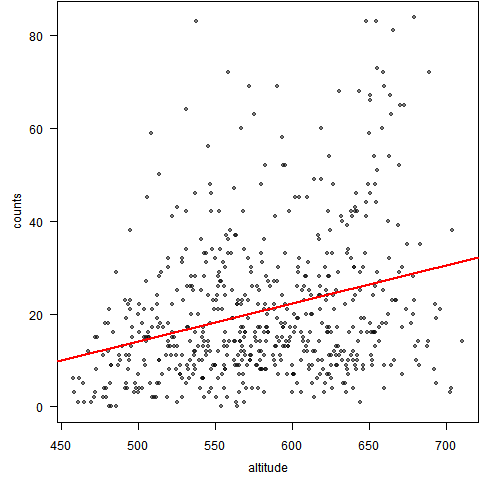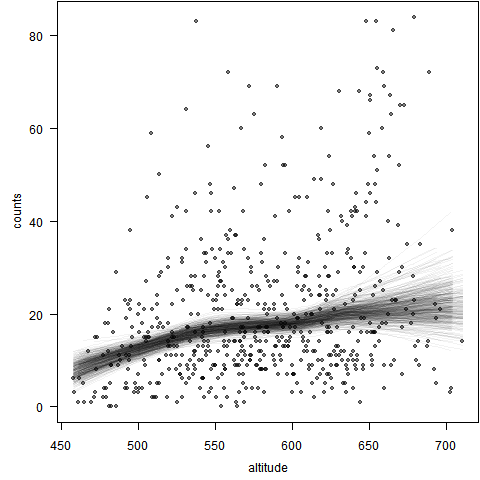LOWESS主要思想是取一定比例的局部数据，在这部分子集中拟合多项式回归曲线，这样我们便可以观察到数据在局部展现出来的规律和趋势；而通常的回归分析往往是根据全体数据建模，这样可以描述整体趋势，但现实生活中规律不总是（或者很少是）教科书上告诉我们的一条直线。我们将局部范围从左往右依次推进，最终一条连续的曲线就被计算出来了。显然，曲线的光滑程度与我们选取数据比例有关：比例越少，拟合越不光滑（因为过于看重局部性质），反之越光滑。

R程序代码：

# 从本站counts.txt文件直接将数据读入R
par(las = 1, mar = c(4, 4, 0.1, 0.1))
plot(x, pch = 20, col = rgb(0, 0, 0, 0.5))
# 取不同的f参数值
for (i in seq(0.01, 1, length = 100)) {
lines(lowess(x$altitude, x$counts, f = i), col = gray(i),
lwd = 1.5)
Sys.sleep(0.15)
}par(las = 1, mar = c(4, 4, 0.1, 0.1), mgp = c(2.5,
1, 0))
plot(x, pch = 20, col = rgb(0, 0, 0, 0.5))
abline(lm(counts ~ altitude, x), lwd = 2, col = "red")set.seed(711) # 设定随机数种子，保证本图形可以重制
par(las = 1, mar = c(4, 4, 0.1, 0.1), mgp = c(2.5,
1, 0))
plot(x, pch = 20, col = rgb(0, 0, 0, 0.5))
for (i in 1:400) {
idx = sample(nrow(x), 300, TRUE) # 有放回抽取300个样本序号
lines(lowess(x$altitude[idx], x$counts[idx]), col = rgb(0,
0, 0, 0.05), lwd = 1.5) # 用半透明颜色，避免线条重叠使得图形看不清
Sys.sleep(0.05)
}
dev.off()